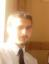# Unknow Function

2 replies to this topic

### #1Berserendo

Berserendo

Newbie

•• Members
•• 1 posts

Posted 13 March 2006 - 10:56 AM

In French :

Bonjour, je viens d'acquerir la Casio Class pad 300+ et j'aimerai savoir si il existe une fonction qui permet de r?soudre cette equation

(-x^3+5x)/(x?+3) = ax + (bx)/(x?+3)

L'objectif est de trouver a et b
Je l'ai fais a la main et j'ai trouv? a = -1 et b = 8. ce sont les resultats et j'en suis sur mais je voudrais tout de meme savoir si la calculatrice a une fonction permettant de resoudre cette ?quationIn english
Hello, i get a classpad 300 yesterday, and i want to know if there is a function who can solv this

(-x^3+5x)/(x?+3) = ax + (bx)/(x?+3)

The target is to find a and b
The result is a = -1 and b - 8 but i want to know who solv it with the classpad

Thanks### #2MicroPro

MicroPro

Casio Overlord

•• Deputy
•• 640 posts
• Gender:Male
• Location:Iran

• Calculators:
Casio ClassPad 300

Posted 14 March 2006 - 08:53 AM

You should write this in the Main application:
solve((-x^3+5x)/(x?+3) = ax + (bx)/(x?+3), a, b )
Also if x is defined you can use the NumSolve application...
(I'm not sure that CP can solve this...)

### #3-Tom-

-Tom-

Casio Freak

•• Members
•• 104 posts
• Location:Poland
• Interests:Tides, Celestial Navigation, Deadreckoning

• Calculators:
Cla\$\$pad 300

Posted 14 March 2006 - 12:28 PM

You should write this in the Main application:
solve((-x^3+5x)/(x?+3) = ax + (bx)/(x?+3), a, b )
Also if x is defined you can use the NumSolve application...
(I'm not sure that CP can solve this...)

I tried... and nothing, with NumSolve also... maybe someone else had luck?

#### 0 user(s) are reading this topic

0 members, 0 guests, 0 anonymous users